# 11.6.3 Formula Editor

### Formula Editor

The formula editor manages the equations of the parametrized input.

Accessing the formula editor

To open the Formula Editor,

• use the button in the table toolbar shown on the left
• click the yellow or red corner of the table cell (a red corner indicates
an error in the formula)
• use the function buttons next to the text boxes in the dialog boxes (see Figure 11.139)

It is also possible to import formulas saved in Excel and to export formulas from RFEM to Excel. For more information about the data exchange with Excel, see Chapter 12.5.2.

Description

In the Formula text box, any formula can be entered manually. When you use the calculator, its results are transferred automatically.

The formula may consist of constant numerical values, parameters, or functions. The result of the equation appears in the field below. Use the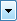button at the end of the Formula line to select an entry from the list of previously entered formulas.

Click the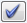button to apply the formula to the table cell or text box of the dialog box. Delete the formula line with the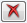button. In case of incorrect entries, formulas are displayed in red in the Formula text box.

Contents of other cells can be used in formulas by means of references.

A reference is introduced by an exclamation point; the reference cell is set in brackets. As shown in the figure above, the content of cell D3 is three times the value of cell D2.

With a prefixed equal sign, you can also enter formulas directly into table cells (e.g. =2.5*PI). If values are used (e.g. =22.1+A*H), they are integrated into the formula in SI units with [m] or [N].

The following functions are available in the calculator of the formula editor:

Table 11.13 Calculator functions
Function Description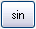Sine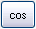Cosine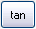Tangent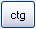Cotangent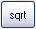Square root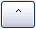Power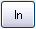Natural logarithm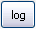Logarithm to the base 10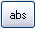Absolute value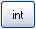Integer, for example int(5.638) = 5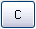Clear formula line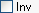Inverse, for example inv√(5) means 52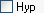Hyperbolic function

The List of Parameters dialog section in the Formula Editor lists all parameters with the current values. To transfer a particular parameter to the Formula line, double-click the entry or select it and use the [Retain] button.

Click the [Edit Parameters] button (see Chapter 11.6.2) to open the parameter list where you can modify or complete the parameters.

Buttons

The buttons available in the formula editor have the following functions.

Table 11.14 Buttons in Edit Formulas dialog box
Button DescriptionApplies formula to table cell or dialog fieldDeletes formula input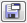Saves content of formula editor as file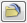Loads a saved file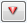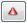Displays or hides calculator and parameter list